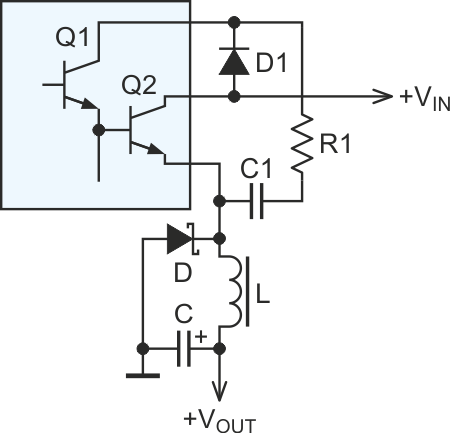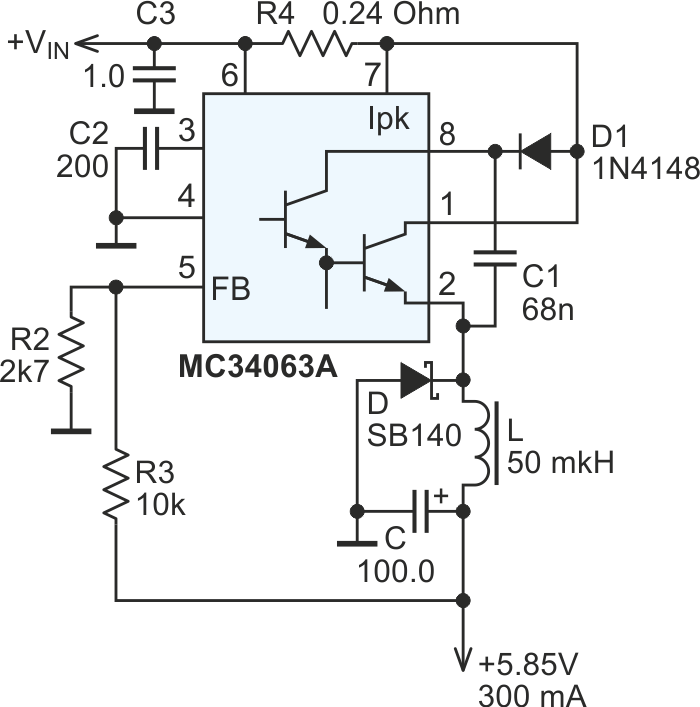# Improve efficiency of low-cost switcher

## ON Semiconductor MC34063

Peter Demchenko

EDN

Low-current switching regulator ICs often use a Darlington as the output switch. The power conversion efficiency in this case can be improved with the help of only two cheap components.

To make this possible, the chip should have a separate pin for the collector of the driver transistor Q1 (Figure 1). At startup, D1 forms a path for the collector current of Q1. Later, D1 and C1 comprise a current-additive rectifier which enhances the collector voltage and current of Q1, hence reducing voltage drop on the closed switch Q2.Figure 1.

Another advantage is the ability to work with lower input voltages. The circuit provides wider input range due to increased voltage on the collector of the driver.

The value of C1 depends on switching frequency. Values in the range of 47 nF to 150 nF are typical.

Resistor R1 may be needed to avoid hard saturation of Q2, or to limit the collector current of Q1, depending on the input voltage and the parameters of Q1. The resistor can be omitted in most cases (i.e., R1 = 0).

An example of this DI concept with the popular MC33063/MC34063 in a buck configuration is shown in Figure 2.Figure 2.

When VIN = 12 V, the above configuration, loaded with 24 Ω, has an efficiency of 85%, and the minimum input voltage is 7.5 V.

Under the same conditions, the standard circuit, without C1 and D1, and with pins 1 and 8 connected, has an efficiency of 78%, and minimum input voltage of 8.2 V.

The same approach can be used with an inverting converter configuration.

EDN